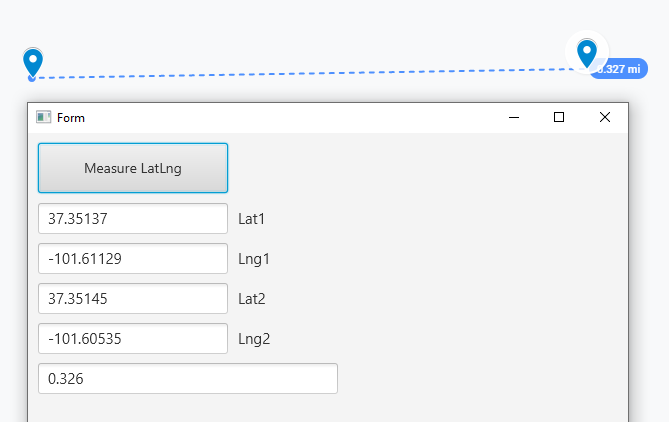# Android Code SnippetMeasure distance between 2 LatLng points

I am working on a mapping application that needs to measure the distance between 2 points, I found a function on Google and convert it to B4A (B4X) code.

K is kilometers
N is nautical miles
M (or anything else) will return the result in miles.
You can add other units into the function and just use math to convert from miles.

decimalPlace = the result will be rounded to X decimal places.

Modify as you wish and enjoy. From my testing, it seems to be very accurate.B4X:
``````Sub MeasureLatLng(lat1 As Double, lng1 As Double, lat2 As Double, lng2 As Double, unit As Char, decimalPlace As Int) As Double
If lat1 = lat2 And lng1 = lng2 Then
Return 0
Else
Dim theta As Double = lng1 - lng2
Dim dist As Double = Sin(lat1 * cPI / 180.0) * Sin(lat2 * cPI / 180.0) + Cos(lat1 * cPI / 180.0) * Cos(lat2 * cPI / 180.0) * Cos(theta * cPI / 180.0)
dist = ACos(dist)
dist = dist / cPI * 180.0
dist = dist * 60 * 1.1515
If unit = "K" Then
dist = dist * 1.609344
Else If unit = "N" Then
dist = dist * 0.8684
End If
dist = Round2(dist, decimalPlace)
Return dist
End If
End Sub``````

•Beja, aminoacid and Almora

#### klaus

##### Expert
Longtime User
To get more precise results you could have used the DistanceTo method from the GPS libary.
B4X:
``````Private Location1, Location2 As Location
Location1.Initialize(Lat1, Lng1)
Location2.Initialize(Lat2, Lng2)
Distance = Location1.DistanceTo(Location2)``````

#### Daica

##### Active Member
To get more precise results you could have used the DistanceTo method from the GPS libary.
B4X:
``````Private Location1, Location2 As Location
Location1.Initialize(Lat1, Lng1)
Location2.Initialize(Lat2, Lng2)
Distance = Location1.DistanceTo(Location2)``````

I read somewhere on the forum that DistanceTo wasn't as accurate, if that's been updated, thats great.
I use my function in B4A as well as B4J server, and I don't think there is a B4J GPS library to use?

#### klaus

##### Expert
Longtime User
Yes, there is no GPS library in B4J.
The code you posted is OK for short distances.
You could simplify your code, replacing:
Sin(lat1 * cPI / 180.0)
by
SinD(lat1)
The same for the others.
All the trigonometric methods with a D at the end, like SinD, CosD etc. work with values in degrees.

Last edited:

Replies
0
Views
1K
Replies
4
Views
1K
Android Question lat lon
Replies
1
Views
731
Android Question Get LatLng on GoogleMaps API
Replies
11
Views
2K
Replies
14
Views
9K